### Surface Areas And Volumes Class 9th Mathematics AP Board Solution

##### Question 1.A closed cylindrical tank of height 1.4 m. and radius of the base is 56 cm. is made up of a thick metal sheet. How much metal sheet is required (Express in square meters)Answer:Given, radius of the base of a cylinder r = 56cm = 0.56mAnd height h = 1.4mTo find the metal sheet required, we need to find the total surface area of the cylinder.Total surface area of the cylinder = 2πr(r + h)= 2 × π × 0.56 × (0.56 + 1.4)= 6.89 ≃ 6.9m2Question 2.The volume of a cylinder is 308 cm3. Its height is 8 cm. Find its later surface area and total surface area.Answer:Given, volume of cylinder = 308 cm3Height = 8cmVolume of the cylinder = πr2h308 = 3.14 × r2 × 8r2 =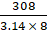r =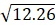r = 3.5cmlateral surface area = 2πrh= 2 × 3.14 × 3.5 × 8= 175.9≃ 176cm2Total surface area = 2πr(r + h)= 2 × π × 3.5 × (3.5 + 8)= 252.89 ≃ 253cm2Question 3.A metal cuboid of dimension 22 cm. × 15 cm. × 7.5 cm. was melted and cast into a cylinder of height 14 cm. What is its radius?Answer:Given, A metal cuboid of dimension 22 cm. × 15 cm. × 7.5 cm. was melted and cast into a cylinder of height 14 cmAccording to the question the volume remains same after casting the cuboid inti cylinder, i.e. lbh = πr2h.22 × 15 × 7.5 = 3.14 × r2 × 14r2 =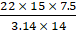r =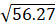r = 7.5cmQuestion 4.An overhead water tanker is in the shape of a cylinder has capacity of 616 litres. The diameter of the tank is 5.6 m. Find the height of the tank.Answer:Given, An overhead water tanker is in the shape of a cylinder has capacity of 616 litresAs 1 litre = 0.001 m3the volume of the cylinder = 0.616 m3.Diameter of the cylinder = 5.6 mRadius of the cylinder =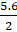= 2.8 mVolume of the cylinder = πr2h.0.616 = 3.14 × 2.82 × h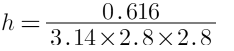h = 0.025 m= 2.5 cmQuestion 5.A metal pipe is 77 cm. long. The inner diameter of a cross section is 4 cm., the outer diameter being 4.4 cm. (see figure) Find its(i) inner curved surface area(ii) outer curved surface area(iii) Total surface area.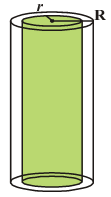Answer:Given, A metal pipe is 77 cm. longInner Diameter = 4cm i.e. r = 2cmOuter diameter = 4.4cm i.e. R = 2.2cm(i) inner curved surface area = 2πrh= 2 × 3.14 × 2 × 77= 967.6cm2(ii) outer curved surface area = 2πRh= 2 × 3.14 × 2.2 × 77= 1063.8cm2(iii) total surface area = 2πrh + 2πrh + 2π(R2 – r2)= 967.6 + 1063.8 + 2 × 3.14 × (2.22 – 22)= 2036.6cm2Question 6.A cylindrical piller has a diameter of 56 cm and is of 35 m high. There are 16 pillars around the building. Find the cost of painting the curved surface area of all the pillars at the rate of 5.50 per 1 m2.Answer:Given, A cylindrical pillar, diameter = 56cm = 0.56m i.e. r = 0.28cmHeight h = 35mNumber of pillars n = 16Curved surface area of a pillar = 2πrh= 2 × 3.14 × 0.28 × 35= 61.54cmCurved surface area of 16 pillars = 16 × 61.54= 984.64cm2Cost of painting = 5.5 × 984.64= 5415.52Question 7.The diameter of a roller is 84 cm and its length is 120 cm. It takes 500 complete revolutions to roll once over the play ground to level. Find the area of the play ground in m2.Answer:Given, diameter = 84cm = 0.84m i.e r = 0.42mLength h = 120cm = 1.20mNumber of revolutions n = 500Area of the playground = n × curved surface area of the roller= 500 × 2πrh= 500 × 2 × 3.14 × 0.42 × 1.2= 1582.56m2Question 8.The inner diameter of a circular well is 3.5 m. It is 10 m deep. Find(i) its inner curved surface area(ii) The cost of plastering this curved surface at the rate of Rs. 40 per m2.Answer:Given, inner diameter of a circular well = 3.5 m i.e. r = 1.75mHeight = 10m(i) curved surface area = 2πrh= 2 × 3.14 × 1.75 × 10= 109.9m2≃ 110cm2(ii) cost of plastering = 40 × 110= 4400Question 9.Find(i) The total surface area of a closed cylindrical petrol storage tank whose diameter 4.2 m. and height 4.5 m.(ii) How much steel sheet was actually used, if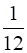of the steel was wasted in making the tank.Answer:(i) Given, diameter = 4.2mRadius = 2.1mHeight = 4.5mTotal surface area = 2πr(r + h)= 2 × 3.14 × 2.1 × (2.1 + 4.5)= 87m2(ii) ifof the steel was wasted in making the tank. The sheet used in making the tank = (1−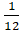) × 87=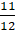× 87= 79.75m2Question 10.A one side open cylinderical drum has inner radius 28 cm. and height 2.1 m. How much water you can store in the drum. Express in litres. (1 litre = 1000 cc.)Answer:Given, r = 28cmh = 2.1m = 210cmto find the capacity of water ,the volume of the cylinder = πr2h= 3.14 × 282 × 210= 516969.6cm31litere = 1000cm3Hence, the water stored =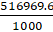= 516.9literesQuestion 11.The curved surface area of the cylinder is 1760 cm.2 and its volume is 12320 cm3. Find its height.Answer:Given, curved surface area = 1760cm2Volume = 12320cm3For finding the height of the cylinder,curved surface area = 1760cm22πrh = 1760rh =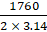rh = 280.25 --------(i)volume = 12320cm3πr2h = 12320cm3r × rh =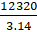r =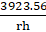from equation (i), r =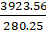r = 14cmhence, rh = 280.25h =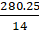h = 20cm

PDF FILE TO YOUR EMAIL IMMEDIATELY PURCHASE NOTES & PAPER SOLUTION. @ Rs. 50/- each (GST extra)

HINDI ENTIRE PAPER SOLUTION

MARATHI PAPER SOLUTION

SSC MATHS I PAPER SOLUTION

SSC MATHS II PAPER SOLUTION

SSC SCIENCE I PAPER SOLUTION

SSC SCIENCE II PAPER SOLUTION

SSC ENGLISH PAPER SOLUTION

SSC & HSC ENGLISH WRITING SKILL

HSC ACCOUNTS NOTES

HSC OCM NOTES

HSC ECONOMICS NOTES

HSC SECRETARIAL PRACTICE NOTES

# 2019 Board Paper Solution

HSC ENGLISH SET A 2019 21st February, 2019

HSC ENGLISH SET B 2019 21st February, 2019

HSC ENGLISH SET C 2019 21st February, 2019

HSC ENGLISH SET D 2019 21st February, 2019

SECRETARIAL PRACTICE (S.P) 2019 25th February, 2019

HSC XII PHYSICS 2019 25th February, 2019

CHEMISTRY XII HSC SOLUTION 27th, February, 2019

OCM PAPER SOLUTION 2019 27th, February, 2019

HSC MATHS PAPER SOLUTION COMMERCE, 2nd March, 2019

HSC MATHS PAPER SOLUTION SCIENCE 2nd, March, 2019

SSC ENGLISH STD 10 5TH MARCH, 2019.

HSC XII ACCOUNTS 2019 6th March, 2019

HSC XII BIOLOGY 2019 6TH March, 2019

HSC XII ECONOMICS 9Th March 2019

SSC Maths I March 2019 Solution 10th Standard11th, March, 2019

SSC MATHS II MARCH 2019 SOLUTION 10TH STD.13th March, 2019

SSC SCIENCE I MARCH 2019 SOLUTION 10TH STD. 15th March, 2019.

SSC SCIENCE II MARCH 2019 SOLUTION 10TH STD. 18th March, 2019.

SSC SOCIAL SCIENCE I MARCH 2019 SOLUTION20th March, 2019

SSC SOCIAL SCIENCE II MARCH 2019 SOLUTION, 22nd March, 2019

XII CBSE - BOARD - MARCH - 2019 ENGLISH - QP + SOLUTIONS, 2nd March, 2019

# HSCMaharashtraBoardPapers2020

(Std 12th English Medium)

HSC ECONOMICS MARCH 2020

HSC OCM MARCH 2020

HSC ACCOUNTS MARCH 2020

HSC S.P. MARCH 2020

HSC ENGLISH MARCH 2020

HSC HINDI MARCH 2020

HSC MARATHI MARCH 2020

HSC MATHS MARCH 2020

# SSCMaharashtraBoardPapers2020

(Std 10th English Medium)

English MARCH 2020

HindI MARCH 2020

Hindi (Composite) MARCH 2020

Marathi MARCH 2020

Mathematics (Paper 1) MARCH 2020

Mathematics (Paper 2) MARCH 2020

Sanskrit MARCH 2020

Sanskrit (Composite) MARCH 2020

Science (Paper 1) MARCH 2020

Science (Paper 2)

Geography Model Set 1 2020-2021

MUST REMEMBER THINGS on the day of Exam

Are you prepared? for English Grammar in Board Exam.

Paper Presentation In Board Exam

How to Score Good Marks in SSC Board Exams

Tips To Score More Than 90% Marks In 12th Board Exam

How to write English exams?

How to prepare for board exam when less time is left

How to memorise what you learn for board exam

No. 1 Simple Hack, you can try out, in preparing for Board Exam

How to Study for CBSE Class 10 Board Exams Subject Wise Tips?

JEE Main 2020 Registration Process – Exam Pattern & Important Dates

NEET UG 2020 Registration Process Exam Pattern & Important Dates

How can One Prepare for two Competitive Exams at the same time?

8 Proven Tips to Handle Anxiety before Exams!